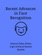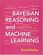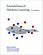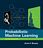Processing ......FreeComputerBooks.com Links to Free Computer, Mathematics, Technical Books all over the World

Pattern Recognition and Machine Learning
Top Free Programming Books 🌠 - 100% Free or Open Source!
• Title Pattern Recognition and Machine Learning
• Author(s) Christopher M. Bishop
• Publisher: Springer (August 17, 2006); eBook (PDF by Microsoft)
• Permission: Link to PDF on the Author's Homepage at Microsoft
• Hardcover 738 pages
• eBook PDF (758 pages)
• Language: English
• ISBN-10: 0387310738
• ISBN-13: 978-0387310732
`Book Description

This is the first textbook on pattern recognition to present the Bayesian viewpoint. The book presents approximate inference algorithms that permit fast approximate answers in situations where exact answers are not feasible.

It uses graphical models to describe probability distributions when no other books apply graphical models to machine learning. No previous knowledge of pattern recognition or machine learning concepts is assumed.

Familiarity with multivariate calculus and basic linear algebra is required, and some experience in the use of probabilities would be helpful though not essential as the book includes a self-contained introduction to basic probability theory.

No previous knowledge of pattern recognition or machine learning concepts is assumed. Familiarity with multivariate calculus and basic linear algebra is required, and some experience in the use of probabilities would be helpful though not essential as the book includes a self-contained introduction to basic probability theory.

• Christopher M. Bishop is the Laboratory Director at Microsoft Research Cambridge, Professor of Computer Science at the University of Edinburgh and a Fellow of Darwin College, Cambridge.
Reviews, Ratings, and Recommendations: Related Book Categories: Read and Download Links:Similar Books:
•Handbook of Digital Face Manipulation and Detection

This open access book provides the first comprehensive collection of studies dealing with the hot topic of digital face manipulation such as DeepFakes, Face Morphing, or Reenactment. It combines the both biometrics and media forensics fields.

•Recent Advances in Face Recognition (Kresimir Delac, et al)

This goal of this book is to provide the reader with the most up to date research performed in automatic face recognition. The chapters presented use innovative approaches to deal with a wide variety of unsolved issues.

•Bayesian Reasoning and Machine Learning (David Barber)

This practical introduction is ideally suited to computer scientists without a background in calculus and linear algebra. You'll develop analytical and problem-solving skills that equip them for the real world. Numerous examples and exercises are provided.

•Gaussian Processes for Machine Learning (Carl E. Rasmussen)

This book provides a long-needed systematic and unified treatment of theoretical and practical aspects of Gaussian Processes (GPs) in machine learning. It deals with the supervised-learning problem for both regression and classification.

•Foundations of Machine Learning (Mehryar Mohri, et al)

This book is a general introduction to machine learning. It covers fundamental modern topics in machine learning while providing the theoretical basis and conceptual tools needed for the discussion and justification of algorithms.

•Understanding Machine Learning: From Theory to Algorithms

This book explains the principles behind the automated learning approach and the considerations underlying its usage. It provides an extensive theoretical account of the fundamental ideas underlying machine learning and the mathematical derivations that transform these principles into practical algorithms.

•Reinforcement Learning: An Introduction, Second Edition

It provides a clear and simple account of the key ideas and algorithms of reinforcement learning that is accessible to readers in all the related disciplines. Focuses on core online learning algorithms, with the more mathematical material set off in shaded boxes.

•Probabilistic Machine Learning: An Introduction (Kevin Murphy)

This book is a comprehensive introduction to machine learning that uses probabilistic models and inference as a unifying approach. It is written in an informal, accessible style, complete with pseudo-code for the most important algorithms.

Book Categories
 :All CategoriesTop Free BooksRecent BooksMiscellaneous BooksComputer EngineeringComputer LanguagesComputer ScienceData Science/DatabasesJava and Java EE (J2EE)Linux and UnixMathematicsMicrosoft and .NETMobile ComputingNetworking and CommunicationsSoftware EngineeringSpecial TopicsWeb Programming
Other Categories